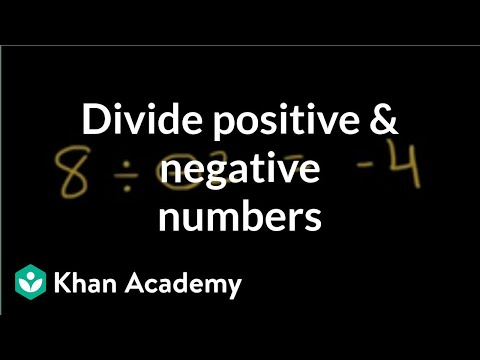Video

# Dividing negative fractions (Full video)

Description: A series of examples of dividing negative numbers. Let's do some examples dividing fractions. Let's say that I have negative 5/6 divided by positive 3/4. Well, we've already talked about when you divide by something, it's the exact same thing as multiplying by its reciprocal.

### Other videos you might be interested in### Comparing rational numbers (Full video)